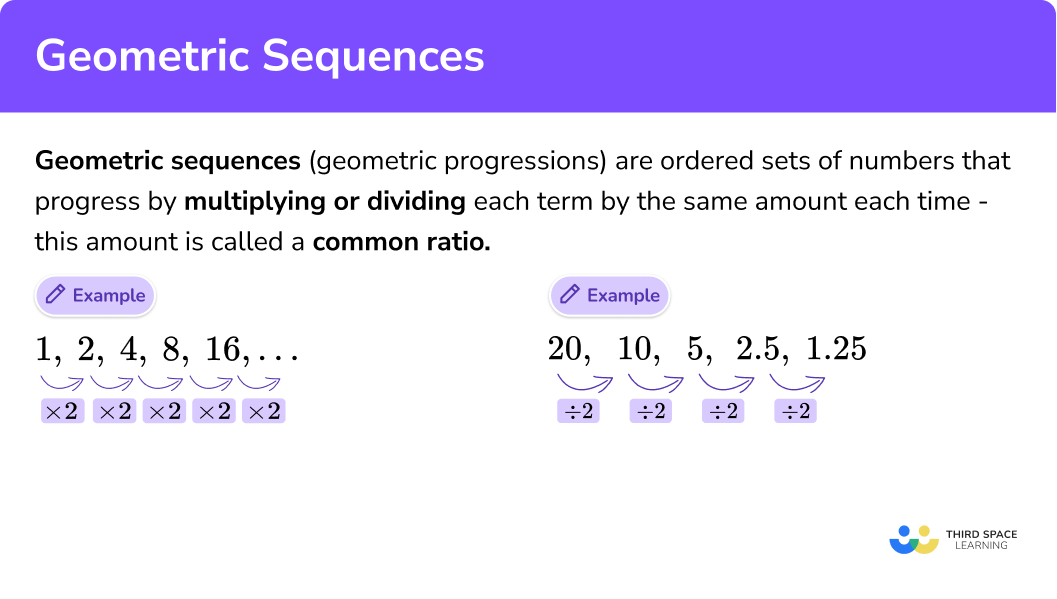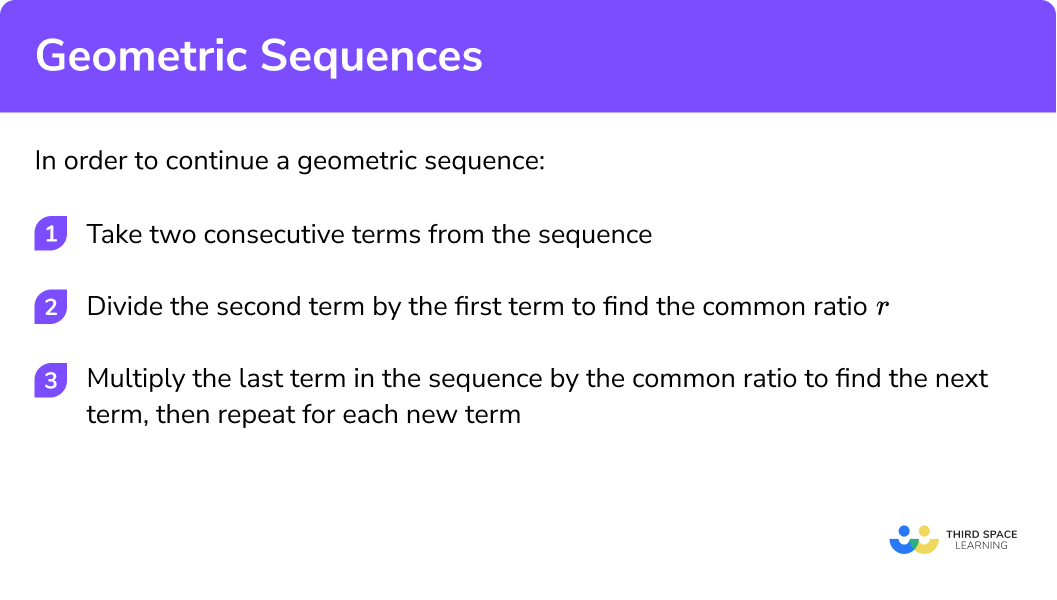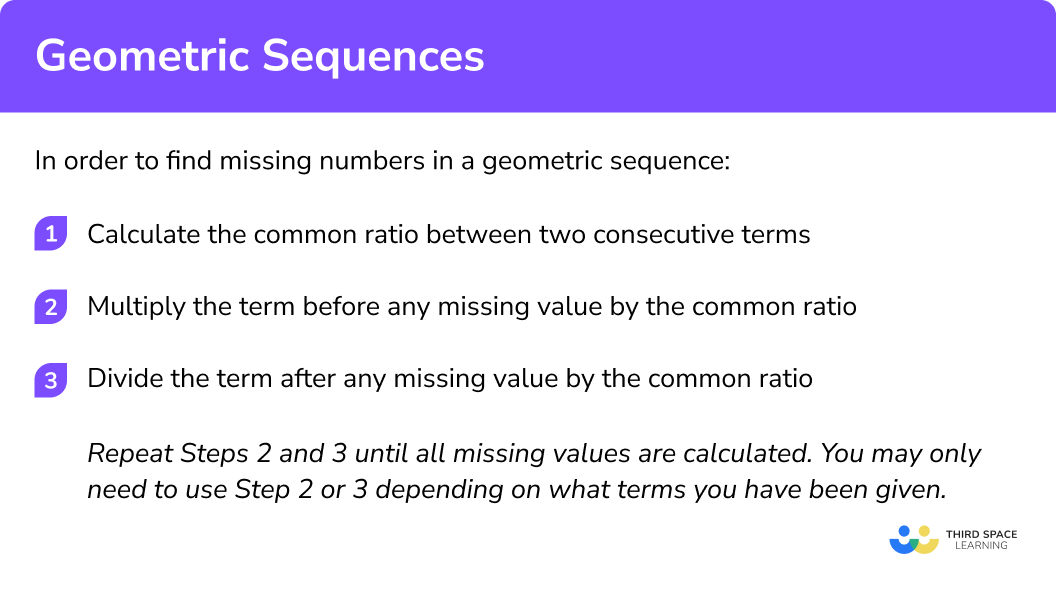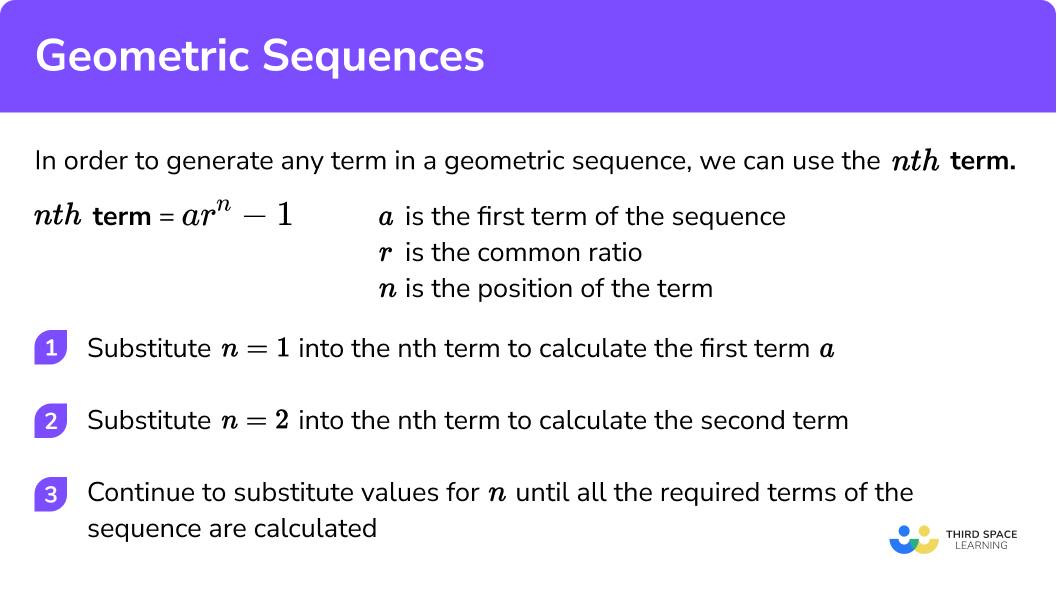GCSE Maths Algebra Sequences

Geometric Sequences

# Geometric Sequences

Here we will learn what a geometric sequence is, how to continue a geometric sequence, how to find missing terms in a geometric sequence, and how to generate a geometric sequence.

At the end, you’ll find geometric sequence worksheets based on Edexcel, AQA, and OCR exam questions, along with further guidance on where to go next if you’re still stuck.

## What is a geometric sequence?

A geometric sequence (geometric progression) is an ordered set of numbers that progresses by multiplying or dividing each term by a common ratio.

If we multiply or divide by the same number each time to make the sequence, it is a geometric sequence.

The common ratio is the same for any two consecutive terms in the same sequence.

Here are a few examples,

First TermTerm-to-Term RuleFirst 5 Terms
3 Multiply by 3 3, 9, 27, 81, 243, ...
5 Multiply by 2 5, 10, 20, 40, 80, ...
-12 Divide by 2 -12, -6, -3, -1.5, -0.75, ...
0.8 Multiply by 5 0.8, 4, 20, 100, 500, ...
\frac{1}{2} Divide by 4 \frac{1}{2}, \; \frac{1}{8}, \; \frac{1}{32}, \; \frac{1}{128}, \; \frac{1}{512} \ldots

### What are geometric sequences?### Geometric sequence formula

The geometric sequence formula is,

Where,

\pmb{ a_{n} } is the n^{th} term (general term),

\pmb{ a_{1} } is the first term,

\pmb{ n } is the term position,

and \pmb{ r } is the common ratio.

We get the geometric sequence formula by looking at the following example,

We can see the common ratio (r) is 2 , so r = 2 .

a_{1} is the first term which is 5 ,

a_{2} is the second term which is 10 ,

and a_{3} is the third term which is 20 etc.

However we can write this using the common difference of 2 ,

### Related lessons on sequences

Geometric sequences is part of our series of lessons to support revision on sequences. You may find it helpful to start with the main sequences lesson for a summary of what to expect, or use the step by step guides below for further detail on individual topics. Other lessons in this series include:

## How to continue a geometric sequence

To continue a geometric sequence, you need to calculate the common ratio. This is the factor that is used to multiply one term to get the next term. To calculate the common ratio and continue a geometric sequence you need to:

1. Take two consecutive terms from the sequence.
2. Divide the second term by the first term to find the common ratio r.
3. Multiply the last term in the sequence by the common ratio to find the next term. Repeat for each new term.

### Explain how to continue a geometric sequence## Continuing a geometric sequence examples

### Example 1: continuing a geometric sequence

Calculate the next three terms for the geometric progression 1, 2, 4, 8, 16,

1. Take two consecutive terms from the sequence.

Here we will take the numbers 4 and 8 .

2 Divide the second term by the first term to find the value of the common ratio, r .

\begin{aligned} r&=8 \div 4 \\\\ r&=2 \end{aligned}

3 Multiply the last term in the sequence by the common ratio to find the next term. Repeat for each new term.

\begin{aligned} 16 \times 2&=32 \\\\ 32 \times 2&=64 \\\\ 64 \times 2&=128 \end{aligned}

The next three terms in the sequence are 32, 64, and 128 .

### Example 2: continuing a geometric sequence with negative numbers

Calculate the next three terms for the sequence -2, -10, -50, -250, -1250, …

Here we will take the numbers -10 and -50 .

r=-50 \div -10

r=5

-1250 \times 5=-6250

-6250 \times 5=-31250

-31250 \times 5=-156250

The next three terms are -6250, -31250, and -156250.

### Example 3: continuing a geometric sequence with decimals

Calculate the next three terms for the sequence 100, 10, 1, 0.1, 0.01,

Here we will take the numbers 0.1 and 0.01 .

\begin{aligned} r&=0.01 \div 0.1 \\\\ r&=0.1 \end{aligned}

\begin{aligned} 0.01 \times 0.1&=0.001\\\\ 0.001 \times 0.1&=0.0001\\\\ 0.0001 \times 0.1&=0.00001\\\\ \end{aligned}

The next three terms in the sequence are 0.001, 0.0001, and 0.00001.

### Example 4: continuing a geometric sequence involving fractions

Calculate the next three terms for the sequence

Here we will take the numbers 5 and 2\frac{1}{2} .

\begin{aligned} r&=2 \frac{1}{2} \div 5\\\\ r&= \frac {1}{2} \end{aligned}

\begin{aligned} \frac{5}{8} \times \frac{1}{2}&=\frac{5}{16} \\\\ \frac{5}{16} \times \frac{1}{2}&=\frac{5}{32} \\\\ \frac{5}{32} \times \frac{1}{2}&=\frac{5}{64} \end{aligned}

The next three terms are

\frac{5}{16}, \frac{5}{32}, and \frac{5}{64} .

### Geometric sequence practice questions – continue the sequence

1. Write the next three terms of the sequence 0.5, 5, 50, 500, …

1000, 5000, 10000950, 1400, 18505000, 50000, 500000550, 555, 560Choose two consecutive terms. For example, 5 and 50 .

Common ratio,

50\div 5=10

500 \times 10=5000, 5000 \times 10=50000, 50000 \times 10=500000

2. Write the next three terms of the sequence 0.04, 0.2, 1, 5, 25, …

50, 100, 200125, 625, 312550, 1000, 2000012.5, 6.25, 3.125Choose two consecutive terms. For example, 5 and 25 .

Common ratio,

25\div 5=5

25 \times 5=125, 125 \times 5=625, 625 \times 5=3125

3. Calculate the next 3 terms of the sequence -1, -3, -9, -27, -81, …

243, 729, 2187-243, -729, -2187243, -729, 2187-27, -9, -3Choose two consecutive terms. For example, -27 and -9 .

Common ratio,

-27\div -9=3

-81 \times 3=-243, -243 \times 3=-729, -729 \times 3=-2187

4. By finding the common ratio, state the next 3 terms of the sequence 640, 160, 40, 10, 2.5 .

0.625, 0.15625, 0.03906251.25, 0.625, 0.312510, 40, 1600.25, 0.025, 0.0025Choose two consecutive terms. For example, 40 and 10 .

Common ratio,

10\div 40=\frac{1}{4} \text{ or } 0.25

2.5 \times 0.25=0.625, 0.625 \times 0.25=0.15625, 0.15625 \times 0.25=0.0390625

5. Work out the common ratio and therefore the next three terms in the sequence 36, 12, 4, \frac{4}{3}, \frac{4}{9}, …

\frac{12}{9}, \frac{36}{9}, \frac{108}{9}\frac{12}{27}, \frac{36}{81}, \frac{108}{243}\frac{4}{12}, \frac{4}{15}, \frac{4}{18}\frac{4}{27}, \frac{4}{81}, \frac{4}{243}Choose two consecutive terms. For example, 12 and 4 .

Common ratio,

4\div 12=\frac{1}{3}

\frac{4}{9} \times \frac{1}{3}=\frac{4}{27}, \frac{4}{27} \times \frac{1}{3}=\frac{4}{81}, \frac{4}{81} \times \frac{1}{3}=\frac{4}{243}

6. Find the common ratio and hence calculate the next three terms of the sequence 1, -1, 1, -1, 1, …

1, 1, 1-1, -1, -11, -1, 1-1, 1, -1Choose two consecutive terms. For example, -1 and 1 .

Common ratio,

-1\div 1=-1

1 \times -1=-1, -1 \times -1=1, 1 \times -1=-1

## How to find missing numbers in a geometric sequence

The common ratio can be used to find missing numbers in a geometric sequence. To find missing numbers in a geometric sequence you need to:

1. Calculate the common ratio between two consecutive terms.
2. Multiply the term before any missing value by the common ratio.
3. Divide the term after any missing value by the common ratio.

Repeat Steps 2 and 3 until all missing values are calculated. You may only need to use Step 2 or 3 depending on what terms you have been given.

### Explain how to find missing numbers in a geometric sequence## Finding missing numbers in a geometric sequence examples

### Example 5: find the missing numbers in the geometric sequence

Fill in the missing terms in the sequence 7, 14, …, …,112 .

\begin{aligned} r&=14 \div 7 \\\\ r&=2 \end{aligned}

14 \times 2=28

112 \div 2 = 56

The missing terms are 28 and 56 .

Note: Here, you could repeat Step 2 by using 28 \times 2 = 56.

### Example 6: find the missing numbers in a geometric sequence including decimals

Find the missing values in the sequence 0.4, …, ..., 137.2, 960.4.

\begin{aligned} r&=960.4 \div 137.2 \\\\ r&=7 \end{aligned}

0.4 \times 7 = 2.8

137.2 \div 7 = 19.6

The missing terms are 2.8 and 19.6 .

### Example 7: find the missing numbers in a geometric sequence when there are multiple consecutive terms missing

Find the missing values in the sequence, -4, ..., …, -108,...

First, we need to find the factor between the two terms, -108 \div -4 = 27 .

To get from -108 to -4 , we jump 3 terms.

This means that -4 has been multiplied by the common ratio three times or -4 \times r \times r \times r = -4r^3 .

\begin{aligned} r^{3}&=27\\\\ r&=3 \end{aligned}

\begin{aligned} -4 \times 3&=-12 \\\\ -12 \times 3&=-36 \\\\ -36 \times 3&=-108 \\\\ -108 \times 3&=-324 \end{aligned}

Note: Term -108 is already given.

The missing terms are -12, -36, and -324.

We don’t need to complete this step.

### Example 8: find the missing numbers in a geometric sequence including mixed numbers

Find the missing values in the sequence

\begin{aligned} r&=13 \frac{1}{2} \div 4 \frac{1}{2}\\\\ r &=\frac{27}{2} \div \frac{9}{2}\\\\ r &=\frac{27}{2} \times \frac{2}{9}\\\\ r &=3 \end{aligned}

13 \frac{1}{2} \times 3=40 \frac{1}{2}

Repeat this step to find the next term.

40 \frac{1}{2} \times 3=121 \frac{1}{2}

4 \frac{1}{2} \div 3=1 \frac{1}{2}

The missing terms in the sequence are

1 \frac{1}{2}, 40 \frac{1}{2}, and 121 \frac{1}{2} .

### Geometric sequence practice questions – find missing numbers

1. Find the missing numbers in the geometric sequence 4, 2, …, 0.5, …

1, 0.21, 0.251, 0.11, 0Choose two consecutive terms. For example, 4 and 2 .

Common ratio,

2\div 4=0.5

2 \times 0.5=1, 0.5 \times 0.5=0.25

2. Find the missing numbers in the sequence -7, -35, …, …, -4375

-175, -875175, 875-4375, -5468751481.7, 2928.3Choose two consecutive terms. For example, -7 and -35 .

Common ratio,

-35\div -7=5

-35 \times 5=-175, -175 \times 5=-875

3. Find the missing terms in the sequence 0.6, …, …, 0.075, 0.0375

0.2, 0.0671.2, 2.40.12, 0.240.3, 0.15Choose two consecutive terms. For example, 0.075 and 0.0375 .

Common ratio,

0.0375\div 0.075=0.5

-35 \times 5=-175, -175 \times 5=-875

4. Calculate the missing terms in the arithmetic sequence 2 \frac{1}{5}, \frac{11}{20}, \frac{11}{80}, \ldots, \ldots

\frac{11}{320}, \frac{11}{1280}\frac{44}{80}, \frac{176}{80}\frac{44}{320}, \frac{176}{1280}\frac{11}{140}, \frac{11}{200}Choose two consecutive terms. For example, \frac{11}{20} and \frac{11}{80} .

Common ratio,

\frac{11}{80} \div \frac{11}{20}=\frac{11}{80} \times \frac{20}{11}=\frac{1}{4}

\frac{11}{80} \times \frac{1}{4}=\frac{11}{320}, \frac{11}{320} \times \frac{1}{4}=\frac{11}{1280}

5. Work out the missing terms in the sequence 3, …, …, 24 .

10, 179, 186, 1212, 183 has been multiplied by the common ratio, r, three times to get 24.

Therefore,

3 \times r \times r \times r=24 \text{ or } 3r^{3}=24 .

Solving the equation,

\begin{aligned} 3r^{3}&=24\\ r^{3}&=8\\ r&=2 \end{aligned}

3 \times 2=6, 6 \times 2=12

6. Work out the missing terms in the sequence 90, …, …, \frac{10}{3} .

\frac{550}{9}, \frac{290}{9}9, 330, 1045, \frac{45}{2}90 has been multiplied by the common ratio, r, three times to get \frac{10}{3}.

Therefore,

90 \times r \times r \times r=\frac{10}{3} \text{ or } 90r^{3}=\frac{10}{3} .

Solving the equation,

\begin{aligned} 90r^{3}&=\frac{10}{3}\\ r^{3}&=\frac{10}{3} \div 90\\ r^{3} = \frac{1}{27} r&=\frac{1}{3} \end{aligned}

90 \times \frac{1}{3}=30, 30 \times \frac{1}{3}=10

## How to generate a geometric sequence

In order to generate a geometric sequence, we need to know the n^{th} term. Using a as the first term of the sequence, r as the common ratio and n to represent the position of the term, the n^{th} term of a geometric sequence is written as ar^{n-1}.

Once we know the first term and the common ratio, we can work out any number of terms in the sequence.

The first term is found when n=1 , the second term when n=2 , the third term when n=3 and so on.

To generate a geometric sequence you need to:

1. Substitute n=1 into the n^{th} term to calculate the first term.
2. Substitute n=2 into the n^{th} term to calculate the second term.
3. Continue to substitute values for n until all the required terms of the sequence are calculated.

### Explain how to generate a geometric sequence## Generating a geometric sequence examples

### Example 9: generate a geometric sequence using the nth term

Generate the first 5 terms of the sequence 4^{n-1} .

When n = 1,\quad 4^{1-1} = 4^{0} = 1 .

When n = 2,\quad 4^{2-1 }= 4^{1} = 4 .

The first 5 terms of the sequence are 1, 4, 16, 64, 256.

### Example 10: generate a geometric sequence using a table

Complete the table for the first 5 terms of the arithmetic sequence 6 \times 2^{n-1}.

### Example 11: generate larger terms in a geometric sequence

A geometric sequence has the n^{th} term \left(\frac{1}{2}\right)^{n} .

Calculate the 1^{st}, 2^{nd}, 10^{th} and 12^{th} terms in the sequence. Express your answers as fractions.

The unknown terms are

1, \frac{1}{4}, \frac{1}{1024}, and \frac{1}{4096} .

### Example 12: generate a geometric sequence with a negative common ratio

Generate the first 5 terms of the geometric sequence 2(- 3)^{n-1} .

When n=1, \quad 2(-3)^{n-1}=2(-3)^{1-1}=2(-3)^{0}=2 \times 1=2 .

When n=3, \quad 2(-3)^{n-1}=2(-3)^{3-1}=2(-3)^{2}=2 \times 9=18 .

When n=4, \quad 2(-3)^{n-1}=2(-3)^{4-1}=2(-3)^{3}=2 \times-27=-54 .

When n=5, \quad 2(-3)^{n-1}=2(-3)^{5-1}=2(-3)^{4}=2 \times 81=162 .

The first 5 terms of the sequence are 2, -6, 18, -54, and 162 .

### Geometric sequence practice questions – generate a sequence

1. Generate the first 5 terms of the sequence 10^{n} .

10, 100, 1000, 10000, 1000001, 10, 100, 1000, 1000010, 20, 30, 40, 50100, 1000, 10000, 100000, 1000000When  n=1, 10^{1}=10 .

When n=2, 10^{2}=100 .

When n=3, 10^{3}=1000 .

When n=4, 10^{4}=10000 .

When n=5, 10^{5}=100000 .

2. Generate the first 5 terms of the sequence 5^{n-1} .

5, 25, 125, 625, 31250.2, 1, 5, 25, 125625, 125, 25, 5, 11, 5, 25, 125, 625When n=1, 5^{1-1}=5^{0}=1 .

When n=2, 5^{2-1}=5^{1}=5 .

When n=3, 5^{3-1}=5^{2}=25 .

When n=4, 5^{4-1}=5^{3}=125 .

When n=5, 5^{5-1}=5^{4}=625 .

3. Generate the first 5 terms of the sequence 4 \times 3^{n-1} .

1, 3, 9, 27, 811, 12, 144, 1728, 207364, 12, 36, 108, 32412, 36, 108, 324, 972When n=1, 4 \times 3^{1-1}=4 \times 3^{0}=4 .

When n=2, 4 \times 3^{2-1}=4 \times 3^{1}=12 .

When n=3, 4 \times 3^{3-1}=4 \times 3^{2}=36 .

When n=4, 4 \times 3^{4-1}=4 \times 3^{3}=108 .

When n=5, 4 \times 3^{5-1}=4 \times 3^{4}=324 .

4. Generate the first 5 terms of the sequence \frac{3^{n}}{6} .

\frac{1}{2}, 1 \frac{1}{2}, 4 \frac{1}{2}, 13 \frac{1}{2}, 40 \frac{1}{2}\frac{1}{2}, \frac{1}{4}, \frac{1}{8}, \frac{1}{16}, \frac{1}{32}\frac{1}{6}, \frac{1}{2}, 1 \frac{1}{2}, 4 \frac{1}{2}, 13 \frac{1}{2}\frac{1}{6}, 1 \frac{1}{2}, 4 \frac{1}{2}, 13 \frac{1}{2}, 40 \frac{1}{2}When n=1, \frac{3^1}{6}= \frac{1}{2} .

When n=2, \frac{3^2}{6}= \frac{9}{6} = 1 \frac{1}{2} .

When n=3, \frac{3^3}{6}= \frac{27}{6} = 4 \frac{1}{2} .

When n=4, \frac{3^4}{6}= \frac{81}{6} = 13 \frac{1}{2} .

When n=5, \frac{3^5}{6}= \frac{243}{6} = 40 \frac{1}{2} .

5. Calculate the 1st, 3rd, 10th and 15th term of the sequence 2^{n}.

2, 4, 8, 162, 8, 1024, 327681, 4, 512, 163844, 16, 2048, 65536When n=1, 2^{1}= 2 .

When n=3, 2^{3}= 8 .

When n=10, 2^{10}= 1024 .

When n=15, 2^{15}= 32768 .

6. Calculate the first 5 terms of the sequence 3 \times (-5)^{n-1}.

3, -15, 75, -375, 18751, -5, 25, -125, 6251, -225, 3375, -50625, 759375-15, 75, -375, 1875, -9375When n=1, 3 \times (-5)^{1-1} = 3 \times (-5)^{0} = 3 .

When n=2, 3 \times (-5)^{2-1} = 3 \times (-5)^{1} = -15 .

When n=3, 3 \times (-5)^{3-1} = 3 \times (-5)^{2} = 75 .

When n=4, 3 \times (-5)^{4-1} = 3 \times (-5)^{3} = -375 .

When n=5, 3 \times (-5)^{5-1} = 3 \times (-5)^{4} = 1875.

### Geometric sequences GCSE exam questions

1. Which sequence is a geometric progression?

1, 3, 6, 10, 15, …. \quad \quad 1, 0.6, 0.2, -0.2, -0.6,….

(1 mark)

1, 3, 9, 27, 81, …..

(1)

2.  Here is a geometric progression,

1, -5, 25, …., 625, …

(a) Find the common ratio.

(b) Work out the fourth term of the sequence.

(3 marks)

(a)

25 \div -5 = – 5

Common ratio = -5

(1)

(b)

25 \times -5

(1)

= -125

(1)

3.  A scientist is studying a type of bacteria. The number of bacteria over the first four days is shown below.

How many bacteria will there be on day 7?

(3 marks)

180 \div 60 = 3

(1)

1620 \times 3 \times 3 \times 3

(1)

43740

(1)

### Common misconceptions

• Mixing up the common ratio with the common difference for arithmetic sequences

Although these two phrases are similar, each successive term in a geometric sequence of numbers is calculated by multiplying the previous term by a common ratio and not by adding a common difference.

• A negative value for r means that all terms in the sequence are negative

This is not always the case as when r is raised to an even power, the solution is always positive.

• The first term in a geometric sequence

The first term is a . With ar^{n-1} , the first term would occur when n = 1 and so the power of r would be equal to 0 . Anything to the power of 0 is equal to 1 , leaving a as the first term in the sequence. This is usually mistaken when a = 1 as it is not clearly noted in the question for example, 2^{n-1} is the same as 1 \times 2^{n-1} .

• Incorrect simplifying of the nth term

For example, 6 \times 3^{n-1} is incorrectly simplified to 18^{n-1} as 6 \times 3 = 18 .

• The difference between an arithmetic and a geometric sequence

Arithmetic sequences are formed by adding or subtracting the same number. Geometric sequences are formed by multiplying or dividing the same number.

## Learning checklist

You have now learned how to:

• Recognise geometric sequences

## Still stuck?

Prepare your KS4 students for maths GCSEs success with Third Space Learning. Weekly online one to one GCSE maths revision lessons delivered by expert maths tutors.

Find out more about our GCSE maths tuition programme.